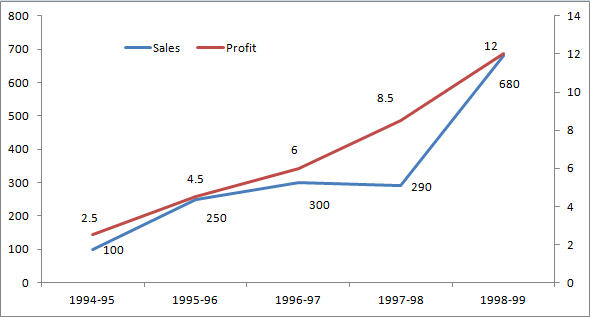### CAT 1999 Question Paper Question 134

Instructions

These questions are based on the situation given below:
The figure below presents sales and net profit, in Rs. Crores, of IVP Ltd for the five years from 1994-95 to 1998-99. During this period, the sales increased from Rs.100 Crores to Rs. 680 Crores. Correspondingly, the net profit increased from Rs. 2.5 Crores to Rs. 12 Crores. Net profit is defined as the excess of sales over total costs.Question 134

# The highest percentage growth in net profit, relative to the previous year, was achieved in

Solution

Percentage growth in net profit in the year 1995-96 is 4.5/2.5 - 1 = 80%
Percentage growth in net profit in the year 1996-97 is 6/4.5 - 1 = 33.33%
Percentage growth in net profit in the year 1997-98 is 8.5/6 - 1 = 41.67%
Percentage growth in net profit in the year 1998-99 is 12/8.5 - 1 = 41.17%

Hence, the percentage growth in net profit is maximum in the year 1995-96